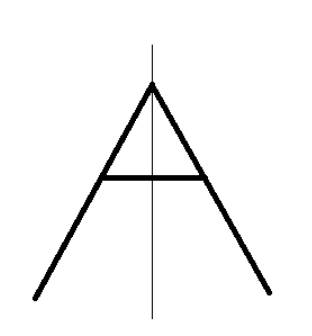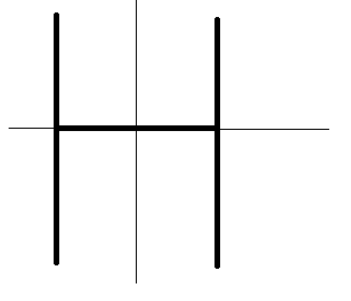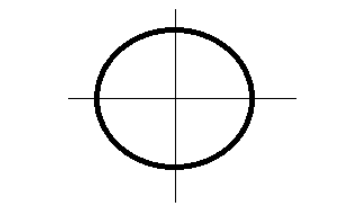QuestionAnswers

# Which of the following alphabets have no linear symmetry?A. AB. LC. HD. O

Hint: In order to solve this problem we need to know that linear symmetry means the object is broken into the same piece or mirror images when it is cut from the middle cut may be horizontal or vertical. Knowing this will solve your problem.

The two parts of the figure coincide, then each part is called the mirror image of the other i.e., the part of the figure on one side of the dotted line falls exactly over the other part which lies on the side. Such a figure is called a symmetrical figure.
The alphabet ‘A’ has linear symmetry that is when it is cut from the middle vertically then it gives us the mirror image of it another part.
A is linearly symmetric, we can show with the help of figure.The alphabet ‘L’ is not linearly symmetric, that is when it is cut from the middle vertically or horizontally it does not give the mirror images of another part.
Now we check the linear symmetry about H.
The alphabet ‘H’ has linear symmetry that is when it is cut from the middle vertically or horizontally then it gives us the mirror image of its other part.
H is linearly symmetric, we can show with the help of figure.The alphabet ‘O’ has linear symmetry that is when it is cut from the middle vertically or horizontally then it gives us the mirror image of its other part.
O is linearly symmetric, we can show with the help of figure.Hence among the four options L is not linearly symmetric.
So, the correct option is B.

Note: In order to solve such problems you need to know that the line of symmetry is the line which passes through the center of the object or any shape. It is considered as the axis or imaginary line of the object. In geometry, you must have learned well about the term symmetry which is defined as a balanced and a proportionate similarity found in two halves of an object, that is, one-half is the mirror image of the other half.